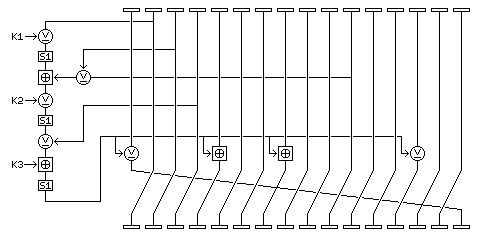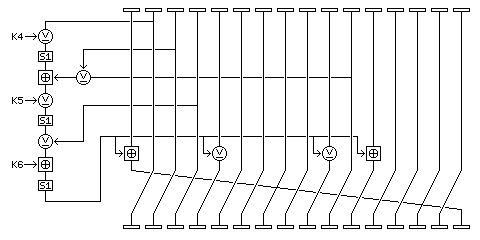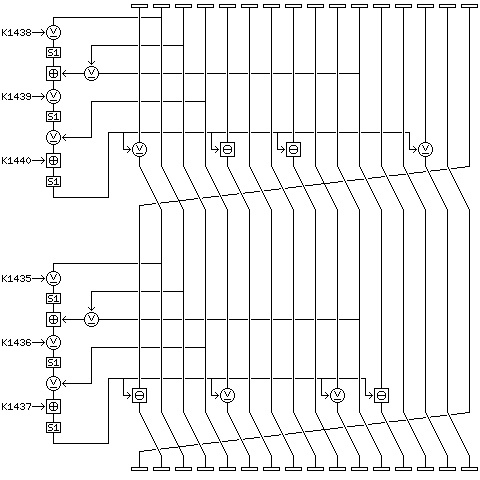[Next] [Up] [Previous] [Index]

Quadibloc 20 is an attempt at a very simple block cipher, inspired by Treyfer, but modified to have a 128-bit block size, to have resistance to slide attacks, and to have faster diffusion in the deciphering direction.

A type A round in Quadibloc 20 looks like the following:and a type B round in Quadibloc 20 looks like the following:There are 30 times 16 rounds in Quadibloc 20, or 480 rounds, and those rounds proceed in the sequence of types:

```A B A B A
```

repeated over and over. Thus, since the number of rounds is a multiple of 5, the cipher is symmetrical in respect of round types. After the last round, the rotate of the block one byte left is omitted.

The operations in a Quadibloc 20 round are as follows:

The block is considered as being composed of sixteen bytes, numbered from 1 through 16 from left to right.

The f-function is calculated as follows:

• A copy of byte 2 is taken.
• This value is XORed with the first subkey byte for the round.
• The result is replaced by its substitute in S-box S1.
• The XOR of bytes 3 and 11 is calculated, and this value is added to the result by means of modulo-256 addition.
• The result then has the second subkey byte for the round XORed to it.
• The result is replaced by its substitute in S-box S1.
• The result is XORed with the value of byte 4.
• The result then has the third subkey byte for the round added to it by means of modulo-256 addition.
• The result is replaced by its substitute in S-box S1.

This f-function is the same in all rounds. The manner in which its output is applied to the block differs in type A rounds and type B rounds.

### In Type A rounds:

Bytes 1 and 14 are modified by being XORed with the f-function output, and bytes 5 and 8 are modified by having the f-function output added to them by means of modulo-256 addition.

### In Type B rounds:

Bytes 1 and 12 are modified by having the f-function output added to them by means of modulo-256 addition, and bytes 5 and 10 are modified by being XORed with the f-function output.

Then, the bytes of the block are rotated one block to the left, in all rounds but the last one.

S-box S1 as used here is the original S-box S1, as defined in the page entitled Euler's Constant and the Quadibloc S-boxes.

### The Key Schedule

The key for Quadibloc 20 is exactly 16 bytes in length, or 128 bits long.

Subkeys are formed from it as follows:

The second subkey byte, in successive rounds, is formed from repeating, over and over, the first seven bytes of the key, with the value of a counter applied to them by modulo-256 addition. The value of the counter begins at zero, and is increased by one each time the seven bytes are used.

The third subkey byte, in successive rounds, is formed from repeating, over and over, the last nine bytes of the key, with the value of a counter XORed to them. The value of the counter begins at zero, and is increased by one each time the nine bytes are used.

The first subkey byte is produced by a somewhat more complicated procedure. It is generated by means of a cycle of 17 bytes; the first 16 bytes are the 16 bytes of the key. The seventeenth byte is the inverse (or one's complement) of the first byte of the key in the first cycle, the second byte of the key in the second cycle, and so on.

Thus, the pattern of subkey bytes is designed to be resistant to slide attacks even for a key consisting entirely of identical bytes. Note that the three different subkey cycles have lengths of 7, 9, and 17, which are not only all relatively prime with each other, but which are also relatively prime with 5, the cycle of round types.

### Decipherment

For decipherment, the first two rounds are shown in the diagram below:### Variations

To improve security, and make it possible to perform decipherment by changing only the key schedule, a modification of the cipher is proposed, to be called Quadibloc 20 S.

In Quadibloc 20 S, after rounds 32, 240, and 448, instead of a byte rotation, a byte transpose is performed, taking the bytes from the order

``` 1  2  3  4  5  6  7  8  9 10 11 12 13 14 15 16
```

to the order

``` 1  5  9 13  2  6 10 14  3  7 11 15  4  8 12 16
```

and as well, while after rounds 1-31 and rounds 33-239, the bytes are rotated one place to the left, after rounds 241-447 and rounds 449-479 the bytes are rotated one place to the right. Also, rounds 241 through 480 are deciphering rounds.

The byte transpose after round 240 does not improve the security of the cipher as much as the other two, due to the existence of the boomerang attack, but it still blocks the slide attack, and, more importantly, it ensures that even if the key schedule somehow turned out to be palindromic, because the order of bytes as presented to the second half of the cipher is completely different, the second half of the cipher cannot undo the encryption performed by the first half of the cipher.